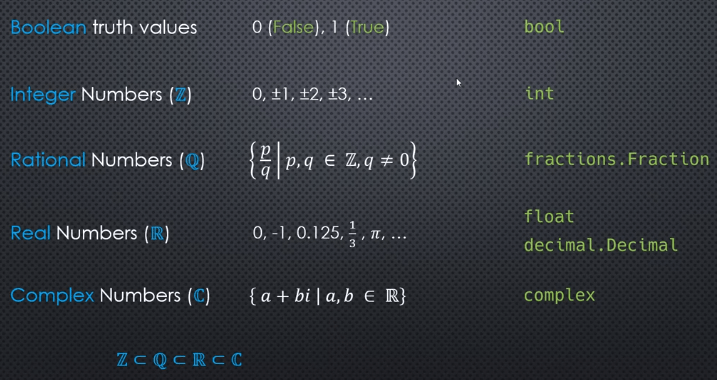## Numeric in Python

### 5 types### int

integers are represented using base-2(binary) digits, not base-10(decimal)

10011(base-2) required 5 bits

##### what’s the range of integer number can be represented using 8 bits?

11111111(base-2) required 8 bits, could represent largest 255

Using 8 bits are able to represent range [-127, 127], since 0 does not require a sign, we can squeeze out an extra number to [-128, 127]

How large an integer can be depends on how many bits are used, some languages(Java, C) provide multiple distinct integer types that use a fixed number of bits. Python doesn’t work that way, the int object uses a variable number of bits, can use 32, 64, 96 bits etc.(increase bits by need)

##### int arithmetic
• int +(-, *, **, //, %) int => int
• int / int => float

### Fraction

represent rational number

### float

represent real number

CPython float is implemented using the C double type which implements the IEEE double-precision binary float, also called binary64

float uses a fixed number of bytes, in standard, CPython/3.6/64bits using 8 bytes(64 bits)##### representation
• decimal• binaryproblem: some numbers do not have a finite representation

• 1/3 can not represent accurate by decimal expansion
• 1/10 can not represent accurate by binary expansion##### Coercing float to integer -> data loss

truncation: math.trunc(), constructor of int() use truncation

floor: math.floor()

ceiling: math.ceil()

round: round()

##### Banker’s Rounding

Read more “Numeric in Python”